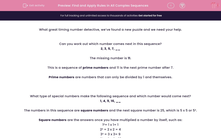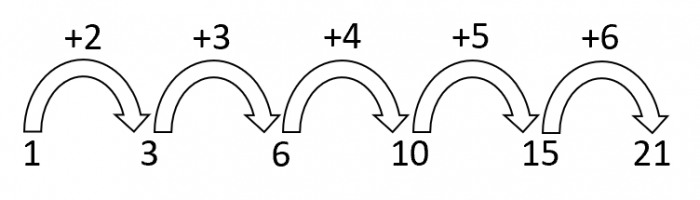# Find and Apply Rules in Complex Sequences

In this worksheet, students will identify missing numbers in a range of complex sequences, including prime, square and triangle numbers.This content is premium and exclusive to EdPlace subscribers.Key stage:  KS 2

Curriculum topic:   Verbal Reasoning

Curriculum subtopic:   Number Sequences

Difficulty level:#### Worksheet Overview

What great timing number detective, we’ve found a new puzzle and we need your help.

Can you work out which number comes next in this sequence?

2, 3, 5, 7, __

The missing number is 11.

This is a sequence of prime numbers and 11 is the next prime number after 7.

Prime numbers are numbers that can only be divided by 1 and themselves.

What type of special numbers make the following sequence and which number would come next?

1, 4, 9, 16, __

The numbers in this sequence are square numbers and the next square number is 25, which is 5 x 5 or 5².

Square numbers are the answers once you have multiplied a number by itself, such as:

1² = 1 x 1 = 1

2² = 2 x 2  = 4

3² = 3 x 3 = 9

4² = 4 x 4 = 16

5² = 5 x 5 = 25

Here is a sequence of numbers called triangle numbers. Can you work out the pattern?

1, 3, 6, 10, 15, 21

In this sequence, the amount added each time is going up by one.The next number in this sequence would be 28 as we need to add 7 next to 21.

It is now your mission to identify the missing numbers in sequences like the ones that we have just looked at.

Make sure you look out for prime numbers, square numbers and triangle numbers.

Write down the relationship between the numbers as you go, it will make it easier to spot a pattern.Good luck number detective!

We are counting on you...

### What is EdPlace?

We're your National Curriculum aligned online education content provider helping each child succeed in English, maths and science from year 1 to GCSE. With an EdPlace account you’ll be able to track and measure progress, helping each child achieve their best. We build confidence and attainment by personalising each child’s learning at a level that suits them.

Get started